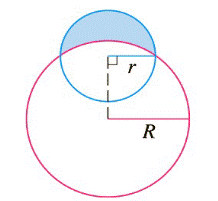Chapter 7.3, Problem 43E

Chapter
Section
Textbook Problem

# Find the area of the crescent-shaped region (called a lune) bounded by arcs of circles with radii r and R. (See the figure.)To determine

To Find: The area of the crescent shaped region

Explanation

Calculation: The Area is obtained by integrating the distance between the two curves with respect to x, from x=r to x=r

From the figure, we have

The larger circle is given by x2+y2=R2 or y=R2x2

The smaller circle is thus described by the equation x2+(yR2x2)2=r2, or y=r2x2+R2r2

A=20r[(r2x2+R2r2)R2x2]dx

=2rR2r2+20r[(r2x2R2x2)]dx

Consider 0ru2x2dx

Let x=usinθ, then dx=ucosθdθ;

u2x2=u2u2sin2θ=u2(1sin2θ)=ucosθ

when x=0,θ=0; when x=r,θ=sin1ru

cosθ=u2x2u0ru2x2dx=0bucosθucosθdθ=obu2

### Still sussing out bartleby?

Check out a sample textbook solution.

See a sample solution

#### The Solution to Your Study Problems

Bartleby provides explanations to thousands of textbook problems written by our experts, many with advanced degrees!

Get Started

#### Find the area of the shaded region.

Single Variable Calculus: Early Transcendentals, Volume I

#### Find the number n such that i=1ni=78.

Single Variable Calculus: Early Transcendentals

#### Evaluate . e + 1

Study Guide for Stewart's Single Variable Calculus: Early Transcendentals, 8th

#### Minimize Subject to C=x+2y 3x+y12x+4y16x0,y0

Finite Mathematics for the Managerial, Life, and Social Sciences